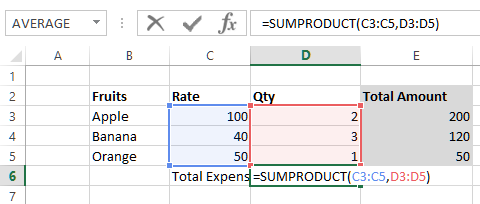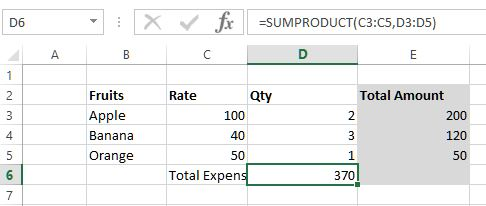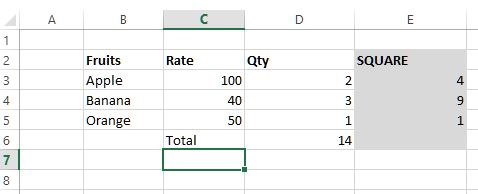# Sumproduct, SumsQ functions in Excel

##### How to sum the product of two ranges and square of given range in Excel?

In previous post, we learnt about Sum, SumIf and SumIfs function in Excel. In this post, we shall learn about SUMPRODUCT, SUMSQ function in MS Excel.

### SumProudct function

SUMPRODUCT function in Excel is used to sum the products (multiplication) of corresponding ranges.

In below example, we want to get the total amount spent, so in D6 cell we have written '=SUMPRODUCT(C3:C5,D3:D5)'  so it does following

1. multiply C3 to D3 and add that value to
2. muliple of C4 to D4 and add that to
3. multiple of C5 to D5
So it basically multiplies the corresponding value of C columns to D columns and gives the result.and here is the result.The benefit we got is that we do not need to have a separate column (We have kept E column just for clarity purpose) to multiple Rate and Qty and then add it.

Thanks for reading, if you liked it do share to your friends and colleagues.

### SumsQ function

SUMSQ function is used to sum the square of the given range. In below example, we have written '=SUMSQ(D3:D5)' formula in D6 cell that squares the value of D3 to D5 cells and add.

Here is the result.The E column is just for reference purpose.

Lik e to SUMSQ we have

1. SUMX2MY2 - used to sums the difference between the squares of two corresponding given ranges
2. SUMX2PY2 - used to return the sum total of the sums of the squares of numbers in two corresponding given ranges
3. SUMXMY2 - used to return the sum of squares of the differences in two corresponding given ranges

As there are not very frequently used so no explanations are provided in this post.

Thanks for reading, if you liked this do share to your friends and colleagues.

Views: 2967 | Post Order: 20